Discrete Mathematics and Combinatorics Commons™

949 Full-Text Articles 1,167 Authors 104,022 Downloads95 Institutions

All Articles in Discrete Mathematics and Combinatorics

949 full-text articles. Page 3 of 35.

Perfect Double Roman Domination Of Trees, 2019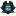East Tennessee State University

Perfect Double Roman Domination Of Trees, Ayotunde Egunjobi

Electronic Theses and Dissertations

See supplemental content for abstract

2019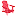University of Lynchburg

Mathematical Models: The Lanchester Equations And The Zombie Apocalypse, Hailey Bauer

Undergraduate Theses and Capstone Projects

This research study used mathematical models to analyze and depicted specific battle situations and the outcomes of the zombie apocalypse. The original models that predicted warfare were the Lanchester models, while the zombie apocalypse models were fictional expansions upon mathematical models used to examine infectious diseases. In this paper, I analyzed and compared different mathematical models by examining each model’s set of assumptions and the impact of the change in variables on the population classes. The purpose of this study was to understand the basics of the discrete dynamical systems and to determine the similarities between imaginary and realistic ...

Series-Parallel Operations With Alpha-Graphs, 2019Valencia College

Series-Parallel Operations With Alpha-Graphs, Christian Barrientos, Sarah Minion

Theory and Applications of Graphs

Among difference vertex labelings of graphs, $\alpha$-labelings are the most restrictive one. A graph is an $\alpha$-graph if it admits an $\alpha$-labeling. In this work, we study a new alternative to construct $\alpha$-graphs using, the well-known, series-parallel operations on smaller $\alpha$-graphs. As an application of the series operation, we show that all members of a subfamily of all trees with maximum degree 4, obtained using vertex amalgamation of copies of the path $P_{11}$, are $\alpha$-graphs. We also show that the one-point union of up to four copies of $K_{n,n}$ is an ...

Potentially Eventually Positive 2-Generalized Star Sign Patterns, 2019Huaiyin Institute of Technology

Potentially Eventually Positive 2-Generalized Star Sign Patterns, Yu Ber-Lin, Ting-Zhu Huang, Xu Sanzhang

Electronic Journal of Linear Algebra

A sign pattern is a matrix whose entries belong to the set $\{+, -, 0\}$. An $n$-by-$n$ sign pattern $\mathcal{A}$ is said to be potentially eventually positive if there exists at least one real matrix $A$ with the same sign pattern as $\mathcal{A}$ and a positive integer $k_{0}$ such that $A^{k}>0$ for all $k\geq k_{0}$. An $n$-by-$n$ sign pattern $\mathcal{A}$ is said to be potentially eventually exponentially positive if there exists at least one real matrix $A$ with the same sign pattern as $\mathcal{A}$ and a nonnegative integer \$t_ ...

2019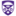The University of Western Ontario

Approximation Algorithms For Problems In Makespan Minimization On Unrelated Parallel Machines, Daniel R. Page

Electronic Thesis and Dissertation Repository

A fundamental problem in scheduling is makespan minimization on unrelated parallel machines (R||Cmax). Let there be a set J of jobs and a set M of parallel machines, where every job Jj ∈ J has processing time or length pi,j ∈ ℚ+ on machine Mi ∈ M. The goal in R||Cmax is to schedule the jobs non-preemptively on the machines so as to minimize the length of the schedule, the makespan. A ρ-approximation algorithm produces in polynomial time a feasible solution such that its objective value is within a multiplicative factor ρ of the optimum ...

Fractional Matching Preclusion For Butterfly Derived Networks, 2019Qinghai University

Fractional Matching Preclusion For Butterfly Derived Networks, Xia Wang, Tianlong Ma, Chengfu Ye, Yuzhi Xiao, Fang Wang

Theory and Applications of Graphs

The matching preclusion number of a graph is the minimum number of edges whose deletion results in a graph that has neither perfect matchings nor almost perfect matchings. As a generalization, Liu and Liu  recently introduced the concept of fractional matching preclusion number. The fractional matching preclusion number (FMP number) of G, denoted by fmp(G), is the minimum number of edges whose deletion leaves the resulting graph without a fractional perfect matching. The fractional strong matching preclusion number (FSMP number) of G, denoted by fsmp(G), is the minimum number of vertices and edges whose deletion leaves the ...

Greatest Common Divisor: Algorithm And Proof, 2019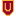University of St. Thomas - Houston

Greatest Common Divisor: Algorithm And Proof, Mary K. Flagg

Number Theory

No abstract provided.

Blackjack: The Math Behind The Cards, 2019Louisiana Tech University

Blackjack: The Math Behind The Cards, Hanna Blanchard

Mathematics Senior Capstone Papers

In this paper the reader will learn about the math behind the cards in the game of Blackjack. Blackjack or “21” has been played around the world with various rules and regulations in both professional and informal environments. The ultimate objective of the game is to receive a total card value of 21, or as close to 21 as possible without exceeding it, from the cards in a player’s hand in order to beat the dealer’s total. The goal of this project is to calculate the probabilities of various hands to determine the best strategies to win 21 ...

Congruence Relations Mod 2 For (2 X 4^T + 1)-Colored Partitions, 2019University of South Carolina

Congruence Relations Mod 2 For (2 X 4^T + 1)-Colored Partitions, Nicholas Torello

Senior Theses

Let p_r(n) denote the difference between the number of r-colored partitions of n into an even number of distinct parts and into an odd number of distinct parts. Inspired by proofs involving modular forms of the Hirschhorn-Sellers Conjecture, we prove a similar congruence for p_r(n). Using the Jacobi Triple Product identity, we discover a much stricter congruence for p_3(n).

Restricted Power Domination And Zero Forcing Problems, 2019Iowa State University

Restricted Power Domination And Zero Forcing Problems, Chassidy Bozeman, Boris Brimkov, Craig Erickson, Daniela Ferrero, Mary Flagg, Leslie Hogben

Mathematics Publications

Power domination in graphs arises from the problem of monitoring an electric power system by placing as few measurement devices in the system as possible. A power dominating set of a graph is a set of vertices that observes every vertex in the graph, following a set of rules for power system monitoring. A practical problem of interest is to determine the minimum number of additional measurement devices needed to monitor a power network when the network is expanded and the existing devices remain in place. In this paper, we study the problem of finding the smallest power dominating set ...

The Knill Graph Dimension From Clique Cover, 2019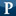Pepperdine

The Knill Graph Dimension From Clique Cover, Evatt Salinger, Dr. Kassahun Betre

Seaver College Research And Scholarly Achievement Symposium

In this paper we prove that the recursive (Knill) dimension of the join of two graphs has a simple formula in terms of the dimensions of the component graphs: dim (G1 + G2) = 1 + dim G1 + dim G2. We use this formula to derive an expression for the Knill dimension of a graph from its minimum clique cover. A corollary of the formula is that a graph made of the arbitrary union of complete graphs KN of the same order KN will have dimension N − 1.

Triangle Packing On Tripartite Graphs Is Hard, 2019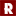University of Kansas

Triangle Packing On Tripartite Graphs Is Hard, Peter A. Bradshaw

Rose-Hulman Undergraduate Mathematics Journal

The problem of finding a maximum matching on a bipartite graph is well-understood and can be solved using the augmenting path algorithm. However, the similar problem of finding a large set of vertex-disjoint triangles on tripartite graphs has not received much attention. In this paper, we define a set of vertex-disjoint triangles as a “tratching.” The problem of finding a tratching that covers all vertices of a tripartite graph can be shown to be NP-complete using a reduction from the three-dimensional matching problem. In this paper, however, we introduce a new construction that allows us to emulate Boolean circuits using ...

Graphs, Random Walks, And The Tower Of Hanoi, 2019Baldwin Wallace University, Berea

Graphs, Random Walks, And The Tower Of Hanoi, Stephanie Egler

Rose-Hulman Undergraduate Mathematics Journal

The Tower of Hanoi puzzle with its disks and poles is familiar to students in mathematics and computing. Typically used as a classroom example of the important phenomenon of recursion, the puzzle has also been intensively studied its own right, using graph theory, probability, and other tools. The subject of this paper is “Hanoi graphs”, that is, graphs that portray all the possible arrangements of the puzzle, together with all the possible moves from one arrangement to another. These graphs are not only fascinating in their own right, but they shed considerable light on the nature of the puzzle itself ...

Asymptotically Optimal Bounds For (T,2) Broadcast Domination On Finite Grids, 2019Williams College

Asymptotically Optimal Bounds For (T,2) Broadcast Domination On Finite Grids, Timothy W. Randolph

Rose-Hulman Undergraduate Mathematics Journal

Let G = (V,E) be a graph and t,r be positive integers. The signal that a tower vertex T of signal strength t supplies to a vertex v is defined as sig(T, v) = max(t − dist(T,v),0), where dist(T,v) denotes the distance between the vertices v and T. In 2015 Blessing, Insko, Johnson, and Mauretour defined a (t, r) broadcast dominating set, or simply a (t, r) broadcast, on G as a set T ⊆ V such that the sum of all signal received at each vertex v ∈ V from the set of towers T ...

A Generalized Newton-Girard Identity, 2019University of Maryland, College Park

A Generalized Newton-Girard Identity, Tanay Wakhare

Rose-Hulman Undergraduate Mathematics Journal

We present a generalization of the Newton-Girard identities, along with some applications. As an addendum, we collect many evaluations of symmetric polynomials to which these identities apply.

Decomposing Graphs Into Edges And Triangles, 2019University of Warwick

Decomposing Graphs Into Edges And Triangles, Daniel Kral, Bernard Lidicky, Taisa L. Martins, Yanitsa Pehova

Mathematics Publications

We prove the following 30 year-old conjecture of Győri and Tuza: the edges of every n-vertex graph G can be decomposed into complete graphs C1,. . .,Cℓ of orders two and three such that |C1|+···+|Cℓ| ≤ (1/2+o(1))n2. This result implies the asymptotic version of the old result of Erdős, Goodman and Pósa that asserts the existence of such a decomposition with ℓ ≤ n2/4.

Dissertation_Davis.Pdf, 2019University of Kentucky

Dissertation_Davis.Pdf, Brian Davis

brian davis

Simplices are the simplest" examples of polytopes, and yet they exhibit much of the rich and subtle combinatorics and commutative algebra of their more general cousins. In this way they are sufficiently complicated --- insights gained from their study can inform broader research in Ehrhart theory and associated fields.

In this dissertation we consider two previously unstudied properties of lattice simplices; one algebraic and one combinatorial. The first is the Poincare series of the associated semigroup algebra, which is substantially more complicated than the Hilbert series of that same algebra. The second is the partial ordering of the elements of the ...

Singular Ramsey And Turán Numbers, 2019University of Haifa-Oranim

Singular Ramsey And Turán Numbers, Yair Caro, Zsolt Tuza

Theory and Applications of Graphs

We say that a subgraph F of a graph G is singular if the degrees d_G(v) are all equal or all distinct for the vertices v of F. The singular Ramsey number Rs(F) is the smallest positive integer n such that, for every m at least n, in every edge 2-coloring of K_m, at least one of the color classes contains F as a singular subgraph. In a similar flavor, the singular Turán number Ts(n,F) is defined as the maximum number of edges in a graph of order n, which does not contain F as a ...

Analysis Of Feast Spectral Approximations Using The Dpg Discretization, 2019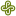Portland State University

Analysis Of Feast Spectral Approximations Using The Dpg Discretization, Jay Gopalakrishnan, Luka Grubišić, Jeffrey S. Ovall, Benjamin Q. Parker

Mathematics and Statistics Faculty Publications and Presentations

A filtered subspace iteration for computing a cluster of eigenvalues and its accompanying eigenspace, known as “FEAST”, has gained considerable attention in recent years. This work studies issues that arise when FEAST is applied to compute part of the spectrum of an unbounded partial differential operator. Specifically, when the resolvent of the partial differential operator is approximated by the discontinuous Petrov Galerkin (DPG) method, it is shown that there is no spectral pollution. The theory also provides bounds on the discretization errors in the spectral approximations. Numerical experiments for simple operators illustrate the theory and also indicate the value of ...

Random Models Of Idempotent Linear Maltsev Conditions. I. Idemprimality, 2019Iowa State University

Random Models Of Idempotent Linear Maltsev Conditions. I. Idemprimality, Clifford Bergman, Agnes Szendrei

Mathematics Publications

We extend a well-known theorem of Murski\v{\i} to the probability space of finite models of a system M of identities of a strong idempotent linear Maltsev condition. We characterize the models of M in a way that can be easily turned into an algorithm for producing random finite models of M, and we prove that under mild restrictions on M, a random finite model of M is almost surely idemprimal. This implies that even if such an M is distinguishable from another idempotent linear Maltsev condition by a finite model A of M, a random search for a ...